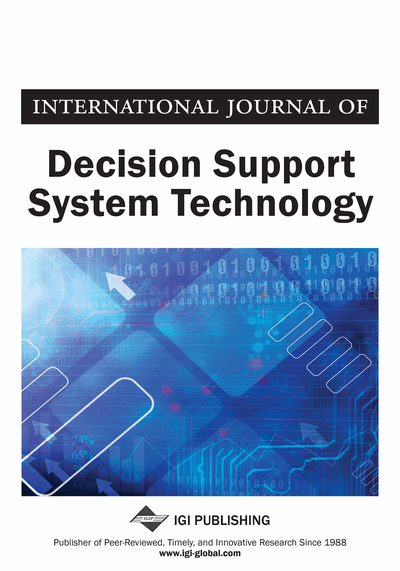# Parallel Hierarchical Pre-Gauss-Seidel Value Iteration Algorithm

Sanaa Chafik (Sultan Moulay Slimane University, Beni Mellal, Morocco), Abdelhadi Larach (Sultan Moulay Slimane University, Beni Mellal, Morocco) and Cherki Daoui (Department of Mathematics, Sultan Moulay Slimane University, Beni Mellal, Morocco)
DOI: 10.4018/IJDSST.2018040101

## Abstract

The standard Value Iteration (VI) algorithm, referred to as Value Iteration Pre-Jacobi (PJ-VI) algorithm, is the simplest Value Iteration scheme, and the well-known algorithm for solving Markov Decision Processes (MDPs). In the literature, several versions of VI algorithm were developed in order to reduce the number of iterations: the VI Jacobi (VI-J) algorithm, the Value Iteration Pre-Gauss-Seidel (VI-PGS) algorithm and the VI Gauss-Seidel (VI-GS) algorithm. In this article, the authors combine the advantages of VI Pre Gauss-Seidel algorithm, the decomposition technique and the parallelism in order to propose a new Parallel Hierarchical VI Pre-Gauss-Seidel algorithm. Experimental results show that their approach performs better than the traditional VI schemes in the case where the global problem can be decomposed into smaller problems.
Article Preview
Top

## 1. Introduction

Markov Decision Processes form a model of stochastic dynamic system, in which, the agent interacts with a deterministic or stochastic environment through a sequence of decision steps. They are used in a wide area of Decision Support Systems Such as: Production Planning, automated control and economics (Chang & al, 2017; Fakoor & al, 2016; Benjaafar & El Hafsi, 2006; Freier & al 2011; Mishra & Singh 2011; Fleischmann & Kuik, 2003; Chan & al, 2006; Boger & al, 2005; Buongiorno & Zhou, 2005; Chen & Lu, 2013; Lewis & al, 2002).

Classical methods are intractable for solving Markov Decision Processes with large state space; it was a great challenge for the researchers, to overcome computational complexity. Many approaches were tried to speed up of the classical solvers: the Value Iteration schemes, the Relaxation Approaches, the hybrid approaches and the general approaches.

In the literature, various Value Iteration schemes have been developed to reduce the computational effort; they represent an improvement of the recursive equations; namely: Value Iteration Pre-Jacobi (VI-PJ) (Blackwell, 1965), Value Iteration Jacobi (VI-J) (Porteus & Totten, 1978), Value Iteration Pre-Gauss-Seidel (VI-PGS) (Porteus, 1975), and Value Iteration Gauss-Seidel (VI-GS) (Kleinman & Kushner, 1971).

The Successive Over-Relaxation (SOR) method (Young, 1954) or the relaxation approaches is a variant of the Gauss–Seidel method for solving a system of linear equations; the SOR uses a relaxation factor for updating the value function at every moment.

The hybridization technique is a kind of the combination algorithms to obtain a new algorithm. A Modified Policy Iteration (M-PI) algorithm, introduced by Puterman and Shin (1978) (Puterman & Shin, 1978), is a combination of the features of the Policy Iteration (PI) algorithm and the Value Iteration algorithm. This modified algorithm completes the update of the value function before performing an update of the policy.

There exist also, general techniques to accelerate most of the algorithms for solving Markov Decision Processes: The action elimination technique introduced by MacQueen (1967) and developed by Porteus (1971) and Porter & al (2008), in which, the number of actions per state can be reduced during each iteration. The decomposition technique introduced by Ross & Varadarajan (1991) for constrained limiting average MDP and used by Abbad & Boustique 2003; Abbad & Daoui (2003); Daoui & Abbad (2007), (2010) in several categories of Markov Decision Processes (average, discounted and weighted MDPs). These techniques are really different flavors of the well-known framework Divide-and-Conquer: (1) partitioning the state space on regions, that is, transforming the initial MDP into small local MDPs, (2) independently solving the local MDPs, and (3) combining the local solutions to obtain an optimal or near-optimal strategy for the global MDP.

In this paper, by combining the advantages of the Pre-Gauss-Seidel (PGS) algorithm, the decomposition technique and the parallelism, the authors propose a new Hierarchical Parallel Value Iteration Pre-Gauss-Seidel algorithm. The experimental results show the performance of our approach in comparison to traditional VI solvers.

This paper is organized as follows: In Section 2, a general introduction to Markov Decision Process under discounted reward criterion will be presented and the VI Pre-Jacobi and VI Pre-Gauss-Seidel schemes will be detailed. In section 3, we present the decomposition technique and the proposed Hierarchical Value Iteration Pre-Gauss-Seidel. In section 4, we discuss the technique of parallelism and we present the improved Hierarchical Parallel VI Pre-Gauss-Seidel algorithm. In section 5, we present the experimental results and in section 6, we discuss a real-world Decision problem in Robotics as application of our approaches. Finally, we conclude and we present our perspectives in future works.

## Complete Article List

Search this Journal:
Reset
Open Access Articles
Volume 12: 4 Issues (2020): 1 Released, 3 Forthcoming
Volume 11: 4 Issues (2019)
Volume 10: 4 Issues (2018)
Volume 9: 4 Issues (2017)
Volume 8: 4 Issues (2016)
Volume 7: 4 Issues (2015)
Volume 6: 4 Issues (2014)
Volume 5: 4 Issues (2013)
Volume 4: 4 Issues (2012)
Volume 3: 4 Issues (2011)
Volume 2: 4 Issues (2010)
Volume 1: 4 Issues (2009)
View Complete Journal Contents Listing